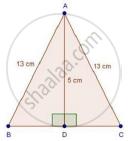Share
Notifications

View all notifications
Books Shortlist
Your shortlist is empty

# Solution for In an Isosceles Triangle Abc, If Ab = Ac = 13 Cm and the Altitude from a on Bc is 5 Cm, Find Bc. - CBSE Class 10 - Mathematics

Login
Create free account

Forgot password?
ConceptApplication of Pythagoras Theorem in Acute Angle and Obtuse Angle

#### Question

In an isosceles triangle ABC, if AB = AC = 13 cm and the altitude from A on BC is 5 cm, find BC.

#### SolutionIn ΔADB, by Pythagoras theorem

AD2 + BD2 = 132

⇒ 25 + BD2 = 169

⇒ BD2 = 169 − 2 = 144

⇒ BD = sqrt144 = 12 cm

In ΔADB and ΔADC

∠ADB = ∠ADC                       [Each 90°]

AB = AC                               [Each 13 cm]

AD = AD                               [Common]

Then, ΔADB ≅ ΔADC               [By RHS condition]

∴ BD = CD = 12 cm               [By c.p.c.t]

Hence, BC = 12 + 12 = 24 cm

Is there an error in this question or solution?

#### Video TutorialsVIEW ALL 

Solution In an Isosceles Triangle Abc, If Ab = Ac = 13 Cm and the Altitude from a on Bc is 5 Cm, Find Bc. Concept: Application of Pythagoras Theorem in Acute Angle and Obtuse Angle.
S